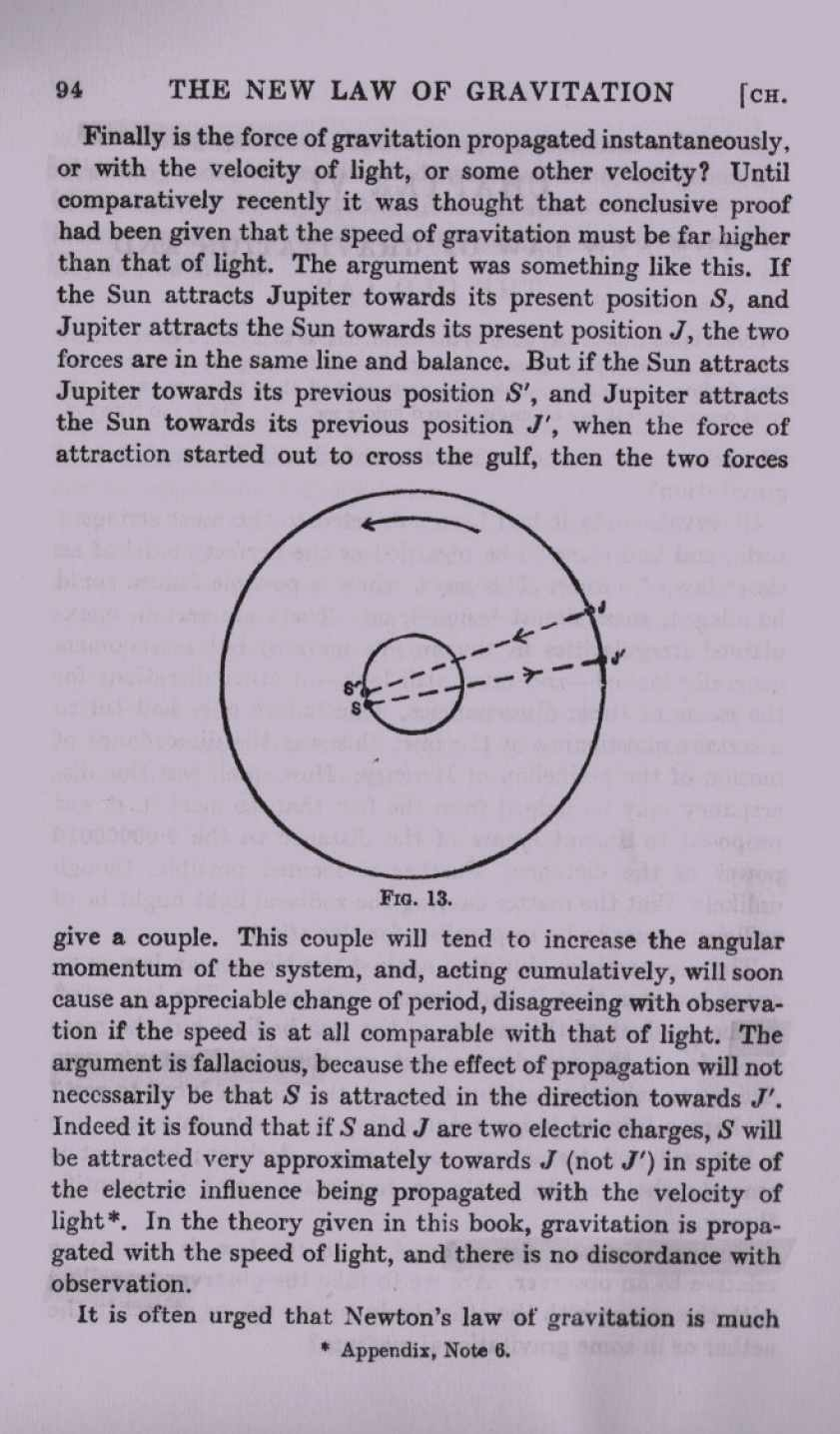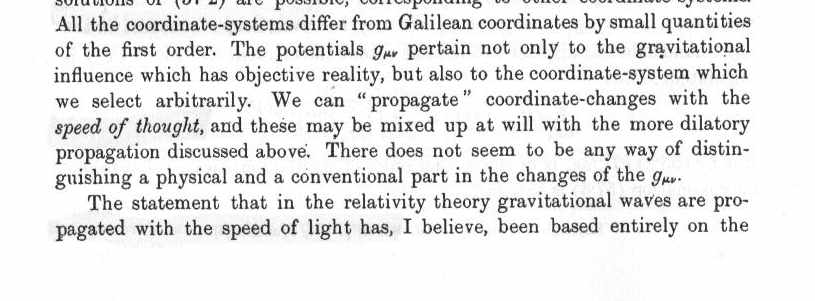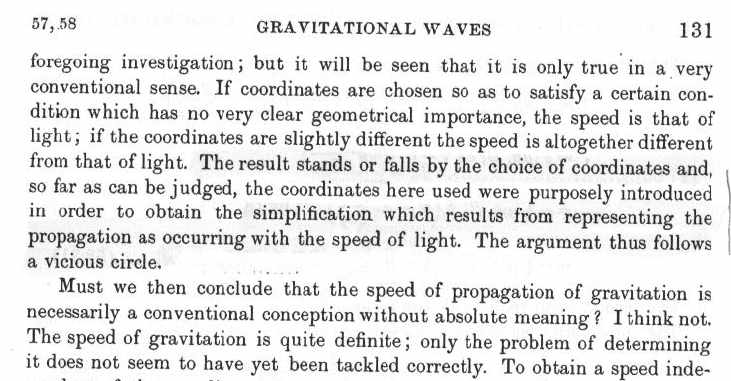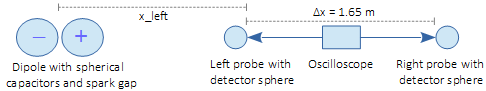Eddington on the Speed of gravity in General Relativity

Arthur Stanley Eddington was a very acco
mplished and brilliant British physicist, mathematician, and astronomer. Shortly after Einstein's theories of Special and General Relativity were published, Eddington authored two books on the subject: Space, Time and Gravitation (1920) and  The Mathematical Theory of Relativity (1923).  The presentation below concerns only what these two books have to say about how fast the force of gravity is propagated.

Eddington points out that it was long thought that the speed of gravitational force was far higher than that of light. The historical argument was that if gravity was propagated at the speed of light, there would be a "force couple" that develops between the present position of Jupiter and the so-called  "retarded position" of Jupiter.  This would result in an alteration of Jupiter's orbit around the Sun which would be easily observed over time.  But no such alteration has been observed. Hence, something is wrong with the argument.

Eddington's belief was that the gravitational force acts much like that of electrical forces between charges. As the footnote referenced on the page below explains "To the first order of vr/C, the denominator is equal to the present distance r, so the expression reduces to e/r in spite of the time propagation. The foregoing formula for the potential was found by Lienard and Wiehert." In other words, the electrical force always points directly to either charge regardless of their motion. There is therefore no applicable "retarded position", and therefore no force couple.  (See The Speed of Electric Fields  for an illustrated explanation.)

Eddington still assumes, strangely, that gravitational force is propagated at the speed of light, and likewise for forces between electric charges.  A. P.  French commits the same error as shown in The Speed of Electric Fields . Evidently, even brilliant minds found instantaneous action-at-a-distance ("non-locality") too inconceivable to take seriously, whether in the 1920s or the 1960s.Space, Time and Gravitation   An Outline of the General Relativity Theory,
A. S. Eddington (1920; reprint 2013) p.94

Eddington's second book (below) shows that the speed of gravity in General Relativity depends on the choice of a coordinate system. In Einstein's General Relativity "the coordinates used were purposely introduced in order to obtain the simplification which results from representing the propagation as occurring with the speed of light." But the choice is arbitrary and "The argument thus follows a vicious circle."

Eddington says that "the speed of gravitation is quite definite".  But in the 1920s no actual laboratory experiments had been done to measure the speed. Hence, the speed was still an open question. Einstein arbitrarily chose the speed of light to  simplify his theory.The Mathematical Theory of Relativity , A. S. Eddington (1923; reprint 2017 by Forgotten Books) p130-131

The take-away from all this is that there is really nothing in Einstein's General Relativity that "proves" or "requires" the speed of gravity to be the same as the speed of light. Indeed, recent investigations indicate that the speed of gravity is very much faster than the speed of light and is probably  instantaneous ("non-local").

https://www.britannica.com/biography/Arthur-Eddington
https://en.wikibooks.org/wiki/Electrodynamics/Lienard-Wiechert_Potentials
http://www.pandualism.com/c/coulomb_experiment.html  :
• Experimental Clarification of Coulomb-Field Propagation

Superluminal information transfer confirmed by simple experiment

Wolfgang G. Gasser  (May, 2016)

Abstract

 A simple experiment has been performed in order to measure propagation speed of the electric field. The results show that the Coulomb interaction propagates substantially faster than at speed of light c.Fig. 1:  Schematic of the experiment

 The experiment uses a spark gap between two conducting spheres acting as capacitors of opposite electric charge. After spark-formation, this rapidly collapsing dipole field is measured by an oscilloscope connected via probes to conducting detector-spheres. Whereas the mutual distance between the detector spheres connected to the oscilloscope remains at Δx = 1.65 m (from left probe tip to right probe tip), different distances from the spark-gap have been measured.

 Id. x_left x_right Δx Δt v = Δx/Δt config_a 1.85 m 3.5 m 1.65 m 3.3 ns 1.7 c config_b 2.6 m 4.25 m 1.65 m 1.1 ns 5.0 c config_c 3.35 m 5 m 1.65 m 1.7 ns 3.2 c config_d 4.85 m 6.5 m 1.65 m 2.1 ns 2.6 c config_e 6.35 m 8 m 1.65 m 2.9 ns 1.9 c config_f 7.85 m 9.5 m 1.65 m 3.8 ns 1.5 c config_g 9.35 m 11 m 1.65 m 4.0 ns 1.4 c

Tab. 1

 The measured propagation speeds v = Δx/Δt from the left to the right detector sphere, with Δt averaged over each five measurements, range from around 1.4 c to 5 c, and show a dependence on the distance from the spark gap.The by far simplest explanation of the experiment is the hypothesis that the Coulomb interaction conforms to Coulomb, who assumed instantaneous interaction at a distance. The dependence of the measured propagation speed on the distance of the measurement setup from the spark gap is explained by dissipative losses and "image charge" complication, leading to electric currents in the ground and the walls.

• Experimental Evidence of Near-field Superluminally Propagating Electromagnetic Fields
William D. Walker(Submitted on 6 Sep 2000)
A simple experiment is presented which indicates that electromagnetic fields propagate superluminally in the near-field next to an oscillating electric dipole source. A high frequency 437MHz, 2 watt sinusoidal electrical signal is transmitted from a dipole antenna to a parallel near-field dipole detecting antenna. The phase difference between the two antenna signals is monitored with an oscilloscope as the distance between the antennas is increased. Analysis of the phase vs distance curve indicates that superluminal transverse electric field waves (phase and group) are generated approximately one-quarter wavelength outside the source and propagate toward and away from the source. Upon creation, the transverse waves travel with infinite speed. The outgoing transverse waves reduce to the speed of light after they propagate about one wavelength away from the source. The inward propagating transverse fields rapidly reduce to the speed of light and then rapidly increase to infinite speed as they travel into the source. The results are shown to be consistent with standard electrodynamic theory.
Comments:17 pages, Presented at Vigier III Symposium: Gravitation and Cosmology, Berkeley, California, USA, August 21-25, 2000Subjects: General Physics (physics.gen-ph); Classical Physics (physics.class-ph) Cite as:arXiv:physics/0009023 [physics.gen-ph] (or  https://arxiv.org/abs/physics/0009023v1     [physics.gen-ph] for this version)  (BF note 1-31-20: The change in speed of the waves within one wavelength of distance sounds a lot like evanescent waves that are being produced along with the usual electromagnetic waves. This should have important practical and theoretical implications.)